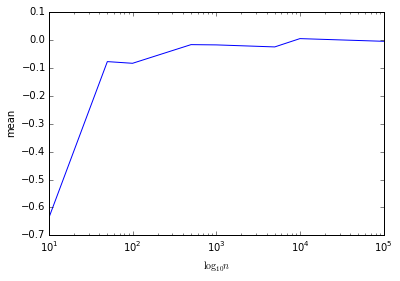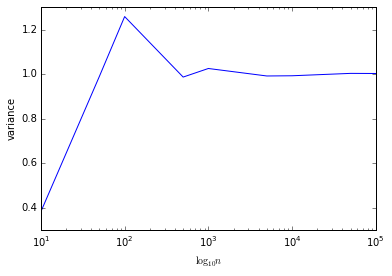# Introduction to Jupyter¶

## Gaussian Process Winter School, Genova, Italy¶

### Neil D. Lawrence¶

Welcome to the Jupyter/IPython notebook! We will be using the Jupyter/IPython notebook for all our lab classes and assignments. It is a really convenient way to interact with data using python. This notebook just alllows us to familiarise ourselves with python and Jupyter.

Python is a generic programming language with 'numerical' and scientific capabilities added on through the numpy and scipy libraries. There are excellent 2-D plotting facilities available through matplotlib. The Jupyter notebook, formerly known as IPython notebook, brings these together in a web based environment that is very convenient for interacting with data.

In my group we switched from using MATLAB to Python a few years ago.

## Importing Libraries¶

The numpy library provides most of the manipulations we need for arrays in python. numpy is short for numerical python, but as well as providing the numerics, numpy provides contiguous array objects. These objects weren't available in the original python. The first step is to import numpy.

In :
import numpy as np


We'll now use numpy to draw samples from a "standard normal". A standard normal is a Gaussian density with mean of zero and variance of one. We'll draw 10 samples from the standard normal. To get help about any command in the notebook simply type that command followed by a question mark.

In :
np.random.normal?


Now let's try sampling from the normal distribution.

In :
X = np.random.normal(loc=0, scale=1, size=(10))


Now let's look at the samples, we can show them using the print command.

In :
print X

[ 1.0024943  -0.51053385  0.732676   -1.84960139  0.16910256 -2.58423356
1.68563628 -0.43361129 -0.1625753  -1.38014833]


## Estimating Mean and Variance¶

We can compute the sample mean by adding all the samples together and dividing by the number of samples.

In :
X.sum()/X.shape

Out:
-0.33307945790849203

Of course we can also estimate the variance, which is easy to write in code as follows

In :
X.var()

Out:
0.17385733568137476

The numpy array object does not behave like a matrix under multiplication. The * sign means element by element multiplication. However, if we construct two matrices as follows and multiply them together., but if we build two matrices and multiply together,

In :
A = np.random.normal(loc=0, scale=1, size=(4, 4))
x = np.random.normal(loc=0, scale=1, size=(4, 1))
print "A=", A
print "x=", x
print "A*x=", A*x

A= [[-1.44642403  1.02088569  0.14406612  0.60383246]
[ 0.22158056 -0.94854781  1.09125545 -0.740506  ]
[ 0.06256555  0.95067385  0.59007773 -0.03108409]
[ 0.88536517  0.11283295 -0.69710017 -0.64578467]]
x= [[ 0.75441334]
[-1.30114054]
[ 1.27996971]
[-0.37354737]]
A*x= [[-1.09120159  0.77016979  0.1086854   0.45553926]
[-0.28830745  1.23419402 -1.4198767   0.96350239]
[ 0.08008201  1.21683373  0.75528163 -0.0397867 ]
[-0.33072583 -0.04214845  0.26039993  0.24123116]]


we still get a result even though the dimensions mismatch. This is because of broadcasting. Python assumes that we want to multiply each column of A by x. This can be convenient, but it can also lead to small bugs. In a lot of mathematical software, if you tried the above operation you'd get a dimension mismatch error.

## Lists and Plotting¶

If we sample from a standard normal, then the true mean and variance of the distribution should be 0 and 1. Of course, the empirical mean and variance won't match the true mean, but let's use matplotlib to plot the convergence towards that value as we increase the number of samples. To do this we are going to use for loops and python lists. We start by creating empty lists for the means and variances. Then we create a list of integers to iterate through. In Python, a for loop always iterates through a list (in some languages this is called a foreach loop, its counterpart the counter for loop only exists by creating a list of integers, see http://en.wikipedia.org/wiki/Foreach_loop#Python). We can use the range command to create the numbers of samples.

In :
# create python 'lists' for the samples, means and variances
samples = [10, 50, 100, 500, 1000, 5000, 10000, 50000, 100000]
means = []
variances = []
for n in samples:
x = np.random.normal(loc=0, scale=1, size=(n))
mean = x.mean()
variance = (x**2).mean() - mean**2
means.append(mean)
variances.append(variance)


### Plotting in Python¶

We'll now plot the variance and the mean against the number of samples. To do this, we need to first convert the samples, varianes and means from Python lists, to numpy arrays.

In :
%matplotlib inline
import matplotlib.pyplot as plt

means = np.asarray(means)
variances = np.asarray(variances)
samples = np.asarray(samples)


Next we need to include the plotting functionality from matplotlib, and instruct the Jupyter notebook to include the plots inline with the notebook, rather than in a different window. First we import the plotting library, matplotlib.

Here we plot the estimated mean against the number of samples. However, since the samples go up logarithmically it's better to use a logarithmic axis for the $x$-axis, as follows.

In :
plt.semilogx(samples, means)
plt.xlabel('$\log_{10}n$')
plt.ylabel('mean')

Out:
<matplotlib.text.Text at 0x10bde5850>We can do the same for the variances, again using a logarithmic axis for the samples. This time, we're going to lavel the x axis using a latex formula.

In :
plt.semilogx(samples, variances)
plt.xlabel('$\log_{10}n$')
plt.ylabel('variance')

Out:
<matplotlib.text.Text at 0x10bde53d0>## Dictionaries¶

Lists are one of the standard datatypes in python. They can contain any datatype.

In :
my_list = ['cat', 7, [3, 'dog']]
print(my_list)

['cat', 7, [3, 'dog']]


For users familiar with java and C++ a list is more akin to a container than an array. Python also provides another container-style data type: the dictionary. Dictionaries are similar to lists but they are indexed by text.

In :
my_dictionary = {'club' : 'Sheffield United', 'stadium' : 'Bramall Lane'}
print(my_dictionary['club'])

Sheffield United


Naturally the two forms can be combined together and you can have dictionaries that contain lists and lists that contain dictionaries.

That's it for the moment, but Jupyter and python have a lot to offer, we'll learn more as we go through the other lab sheets.